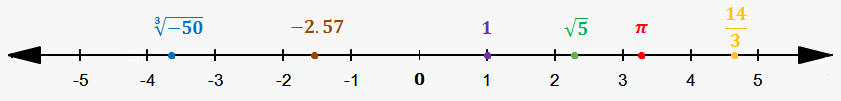# Real Numbers

Real numbers are rational numbers plus irrational numbers.

Since rational numbers include integers and fractions, then real numbers include:

• integers {..., -5,-4,-3,-2,-1,0,1,2,3,4,5,...}
• fractions (proper, improper, mixed numbers)
• irrational numbers (like $\sqrt{{{2}}}$, ${\sqrt[{{3}}]{{-{15}}}}$, $\pi$, ${e}$ etc.)

A set of real numbers, i.e. a group of real numbers, is denoted by $\mathbb{R}$.

As we already saw, all these numbers can be placed on a number line, which is called real number line.Real numbers are called "real", because they are used in math to represent something:

• $\pi$ is used to describe the ratio of circumference of any circle and its diameter
• $\sqrt{{{2}}}$ is used to describe diagonal of unit square
• $\frac{{5}}{{7}}$ is used to describe 5 pieces of cake, that is divided into 7 pieces.